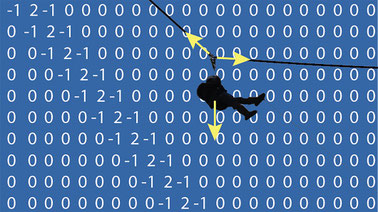## Monday, July 20, 2020

### Differential Equations: Linear Algebra and NxN Systems of Differential EquationsDifferential equations are the mathematical language we use to describe the world around us. Most phenomena can be modeled not by single differential equations, but by systems of interacting differential equations.&nbsp; These systems may consist of many equations. In this course, we will learn how to use linear algebra to solve systems of more than 2 differential equations. We will also learn to use MATLAB to assist us.We will use systems of equations and matrices to explore: The original page ranking systems used by Google, Balancing chemical reaction equations, Tuned mass dampers and other coupled oscillators, Three&nbsp;or more species competing for resources in an ecosystem, The trajectory of a rider on a zipline. The five modules in this series&nbsp;are being offered as an XSeries on edX. Please visit the Differential Equations&nbsp;XSeries Program Page to learn more and to enroll in the modules.*Zipline photo by teanitiki on Flickr (CC BY-SA 2.0)